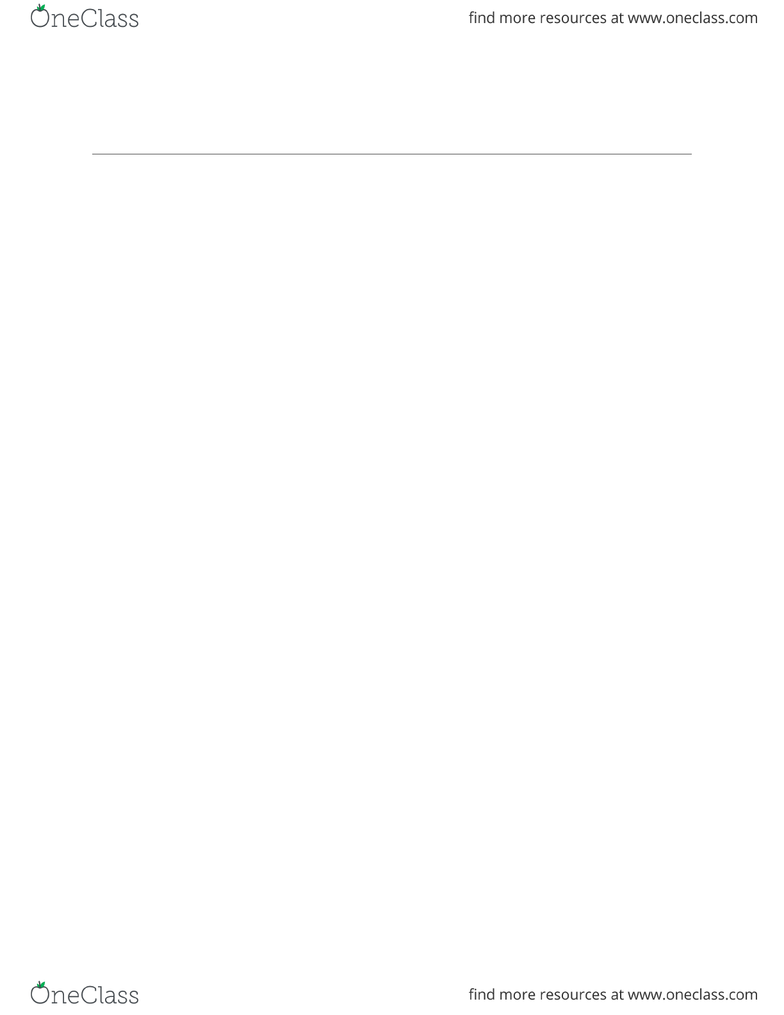Textbook Notes (270,000)
CA (160,000)
UVic (500)
COM (100)
Chapter 3

COM 316 Chapter Notes - Chapter 3: Contribution Margin, Income Statement, Fixed Cost

Department
Commerce
Course Code
COM 316
Professor
Klatt Margaret
Chapter
3

Page:
of 2Wednesday, January 13, 2016
COM 316! !
COST BEHAVIOUR: ANALYSIS & USE
-Describe how fixed and variable costs behave and how to use them to predict costs
Variable costs - total dollar amount varies in direct proportion to changes in the activity
level, remains constant if expressed on a per unit basis
Fixed costs - remain constant within the relevant range of activity, average fixed cost
become progressively smaller as the level of activity increases
-Analyze mixed costs using various approaches
Account analysis - a method for analyzing cost behaviour in which each account under
consideration is classified as either variable or fixed based on the analyst’s prior
knowledge of how the cost in the account behaves (fixed is calculated as sum of fixed
costs, variable is calculated as division of sum of cost of variable account by the total
activity) * useful when past info is available on activity and costs
Engineering approach - a detailed analysis of cost behaviour based on an industrial
engineer’s evaluation of the input required to carry out a particular activity and of the
prices of those inputs * useful when no past experience is available on activity and cost
-Prepare an income statement using the contribution format
Contribution approach - an income statement format where costs are separated into
variable and fixed categories
Cost structure: the relative proportion of fixed, variable and mixed costs found in an
organization
Activity base: a measure of whatever causes a variable cost to be incurred. For example, the
total cost of direct materials in a bicycle manufacturing company will increase as the number of
bicycles produced increases. Therefore, the number of bicycles produced is an activity base for
explaining the total cost of direct materials
VARIABLE COST TYPES
True variable costs: direct materials, amount used varies in direct proportion to the level of
production activity
1
Wednesday, January 13, 2016
Step variable cost: a cost (such as the cost of a maintenance worker) that is obtainable only in
large amounts and that increases and decreases only in response to fairly wide changes in the
activity level.
Curvilinear costs: costs that show a curved relationship between cost and activity rather than a
straight line relationship - can be reasonably approximated with a straight line only within the
band of activity known as the relevant range (where assumptions made about cost behaviour are
valid).
FIXED COST TYPES
Committed fixed costs: fixed costs that are difficult to adjust in the short term and that relate to
the investment in facilities, equipment, and the basic organizational structure of a firm - long
term planning horizon
Discretionary (managed) fixed costs: fixed costs arising from annual decisions by management
to spend in certain areas, such as advertising and research - short term planning horizon
(annually)
Mixed cost equation
Y = a + bX
Y= total mixed cost/ a = total fixed cost/ b = variable cost per unit of activity/ X = activity level
Linear cost behaviour: cost behaviour where the relationship between cost and activity can be
reasonably approximated by a straight line
High low method: a method of separating a mixed cost into its fixed and variable elements by
analyzing the change in cost between the high and low levels of activity
High low formula Variable cost = slope of the line = rise / run = y2 -y1 / x2 - x1
Determining fixed cost element = total cost - variable cost element
Contribution margin: the amount remaining from sales revenues after all variable expenses
have been deducted
2